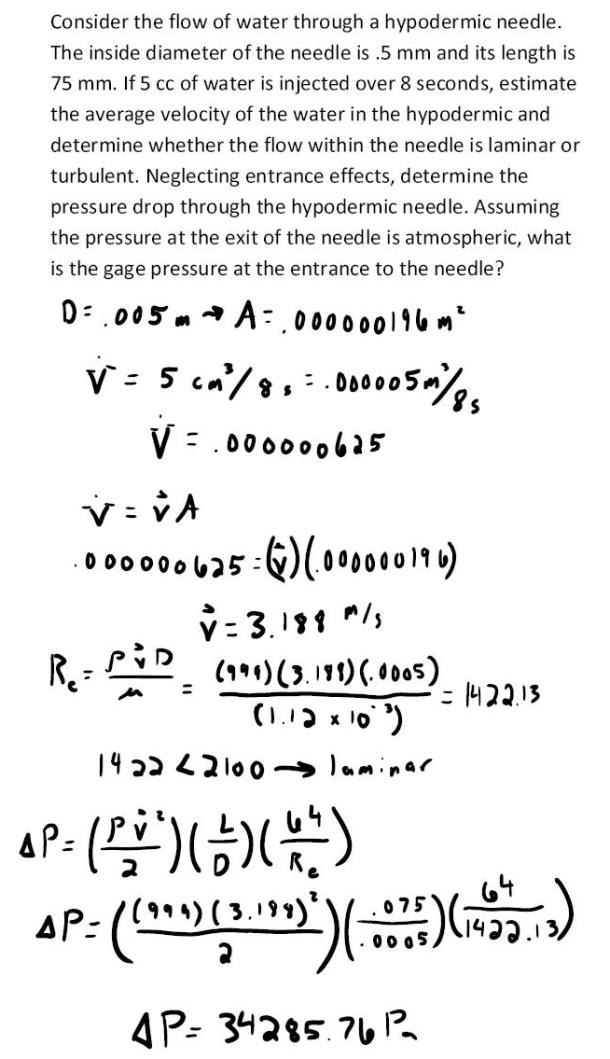fluid mechanics math problems engineering equations fluid mechanics basic mechanics school homework engineering math fluids formulas fluid mechanics problems fluid mechanics problem solutions to fluids problems full solution fluid mechanics engineering fluids problem solution fluid mechanics math problems engineering equations fluid mechanics basic mechanics school homework engineering math fluid mechanics formulas fluid problems fluid mechanics problem solutions to fluid mechanics problems full solution fluid mechanics engineering fluid mechanics problem solution
fluid mechanics math problems engineering equations fluid mechanics basic mechanics school homework engineering math fluids formulas fluid mechanics problems fluid mechanics problem solutions to fluids problems full solution fluid mechanics engineering fluids problem solution fluid mechanics math problems engineering equations fluid mechanics basic mechanics school homework engineering math fluid mechanics formulas fluid problems fluid mechanics problem solutions to fluid mechanics problems full solution fluid mechanics engineering fluid mechanics problem solution
Highalphabet Home Page fluid mechanics solutions fluids math solved Fluid Mechanics Page 1
Consider the flow of water through a hypodermic needle. The inside diameter of the needle is .5 mm and its length is 75 mm. If 5 cc of water is injected over 8 seconds, estimate the average velocity of the water in the hypodermic and determine whether the flow within the needle is laminar or turbulent. Neglecting the entrance effects, determine the pressure drop through the hypodermic needle. Assuming the pressure at the exit of the needle is atmospheric, what is the gage pressure at the entrance to the needle?Consider the flow of water through a hypodermic needle. The inside diameter of the needle is .5 mm and its length is 75 mm. If 5 cc of water is injected over 8 seconds, estimate the average velocity of the water in the hypodermic and determine whether the flow within the needle is laminar or turbulent. Neglecting the entrance effects, determine the pressure drop through the hypodermic needle. Assuming the pressure at the exit of the needle is atmospheric, what is the gage pressure at the entrance to the needle?